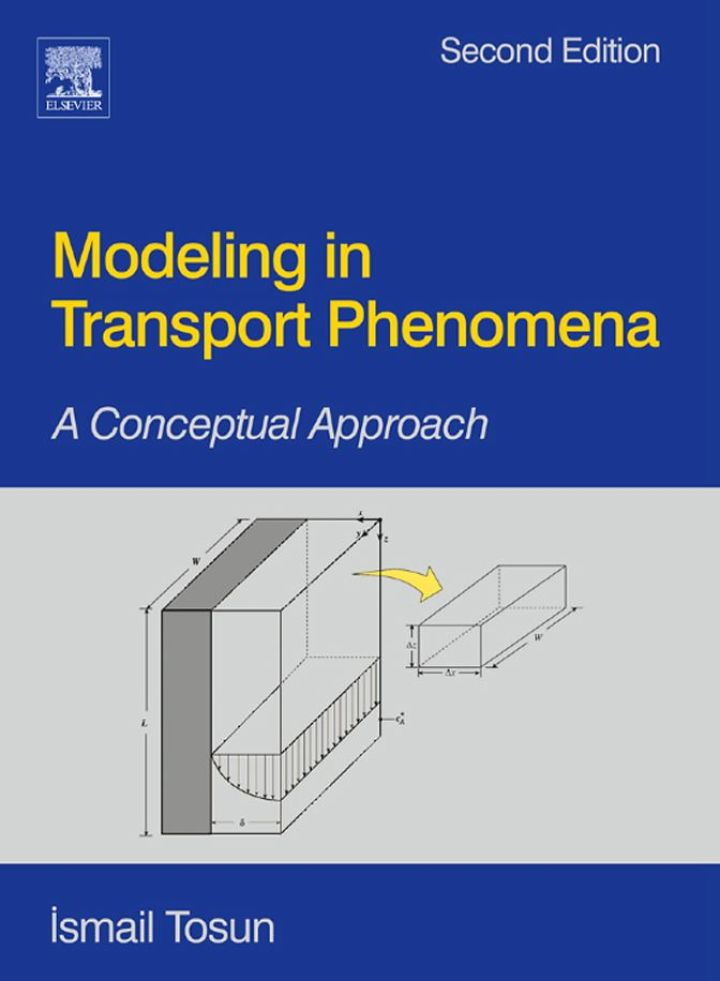# Modeling in Transport Phenomena: A Conceptual Approach

## Modeling in Transport Phenomena: A Conceptual Approach

By Tosun, Ismail

### Rent or Buy eTextbook

365 days
\$93.95
180 days
\$42.28
150 days
\$38.52
120 days
\$36.65
90 days
\$33.83
30 days
\$28.19
Expires on Apr 16th, 2022
\$93.95

Publisher List Price: \$109.99
Savings: \$16.04

Modeling in Transport Phenomena, Second Edition presents and clearly explains with example problems the basic concepts and their applications to fluid flow, heat transfer, mass transfer, chemical reaction engineering and thermodynamics. A balanced approach is presented between analysis and synthesis, students will understand how to use the solution in engineering analysis. Systematic derivations of the equations and the physical significance of each term are given in detail, for students to easily understand and follow up the material.
There is a strong incentive in science and engineering to understand why a phenomenon behaves the way it does. For this purpose, a complicated real-life problem is transformed into a mathematically tractable problem while preserving the essential features of it. Such a process, known as mathematical modeling, requires understanding of the basic concepts. This book teaches students these basic concepts and shows the similarities between them. Answers to all problems are provided allowing students to check their solutions. Emphasis is on how to get the model equation representing a physical phenomenon and not on exploiting various numerical techniques to solve mathematical equations.

* A balanced approach is presented between analysis and synthesis, students will understand how to use the solution in engineering analysis.
* Systematic derivations of the equations as well as the physical significance of each term are given in detail
* Many more problems and examples are given than in the first edition - answers provided

Subject: Physical & Earth Sciences -> Chemistry -> Organic Chemistry2nd edition
Publisher: Elsevier S & T 07/2007
Imprint: Elsevier Science
Language: English
Length: 628 pages

ISBN 10: 0444530215
ISBN 13: 9780444530219
Print ISBN: 9780444530219

Live Chats